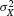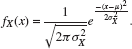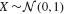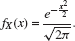# APPENDIX BA COLLECTION OF USEFUL PROBABILITY DISTRIBUTIONS

## B.1 UNIVARIATE DISTRIBUTIONS

• Gaussian distribution: X is a Gaussian random variable with mean μ and varianceif it has the following probability density function (pdf):When X is such a Gaussian random variable, we denote it as. Obviously in this case,and.

• Standard Gaussian distribution: X is a standard Gaussian (or standard normal) random variable if. The pdf of a standard Gaussian random variable X isIn other words,and Var(X) = 1.

• Lognormal distribution: X is a lognormal random variable with parameter μ and σ2 if it has the following pdf:

If X = eY where , then X is lognormal distributed.

• Exponential distribution ...

Get Signal Processing for Cognitive Radios now with the O’Reilly learning platform.

O’Reilly members experience books, live events, courses curated by job role, and more from O’Reilly and nearly 200 top publishers.